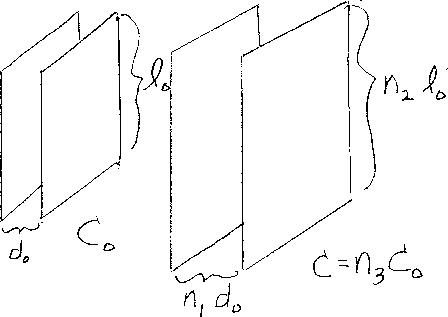Problem B13: In the figure above there are two capacitors, each with square plates. In the one on the left, the plates are separated by a distance d0, and each square plate has a side of length l0. The capacitance of the left capacitor is C0. For the capacitor on the right, the plates are separated by a distance of n1d0, and each square plate has a side of length n2l0. If the capacitance of the right capacitor is n3C0, what is n3 ?n1 = n2 = Input n3:
If you are currently in my class, you can record your grade by entering your name and student ID number (without the leading zeros) below and clicking on "record grade".
 First Name = Last Name = ID = Problem: